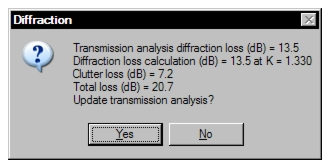# Diffraction

## Diffraction Overview

All diffraction algorithms are deterministic. A terrain profile is required and the calculations are based on the profile geometry. An accurate representation of the effective earth radius is achieved by adding temporary points to the profile using linear interpolation so that the minimum spacing between points is one percent of the profile length.

All calculations are inhibited if either antenna height is zero.

Diffraction loss calculation is saved in the Pathloss data file; however, the results are automatically erased whenever a parameter is changed, which would invalidate these results. These parameters include:

• terrain profile elevations path length
• structures (locations and heights) frequency
• polarization antenna heights
• earth radius factor K the diffraction algorithmThe diffraction loss is not automatically transferred to the Transmission Analysis module. Many operations are carried out in the diffraction section which are not applicable to the transmission path. These include the calculation of diffraction loss as a function of the earth radius factor (K) and analysing the loss from a reflection point to an antenna. If the Transmission Analysis diffraction loss entry is different than the current diffraction loss calculation, you will be prompted to use the calculated value.